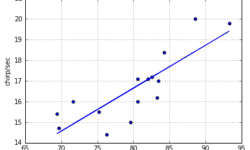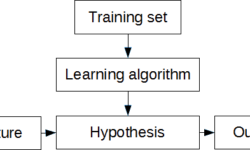## Linear regression – learning algorithm with PythonIn this post, we are going to demystify the learning algorithm of linear regression. We are going to analyze the simplest univariate case with single feature X wherein the previous example was temperature and output was cricket chirps/sec. Let’s use the same data with crickets to build learning algorithm and see if it produces a similar hypothesis as in excel. As you may already know from this example, we need to find linear equation parameters θ0 and θ1, to fit line most optimally on the given data set: y = θ0 + θ1 x x here is a feature (temperature) and y – output value (chirps/sec). So how we are going to find parameters θ0 and θ1? The whole point of the learning algorithm is doing this iteratively. We need to find optimal θ0, θ1 parameter values, so that approximation line error from the plotted training set is minimal. By doing successive corrections to randomly selected parameters we can find an optimal solution. From statistics, you probably know the Least Mean Square (LMS) algorithm. It uses gradient-based method of steepest descent.

## Simplest machine learning algorithm – linear regression with excelSome may say that linear regression is more statistical problem. And this is truth at some level. But when problem is solved from machine learning perspective, things gets easier especially when moving towards more complex problems. First of all lets understand few important terms. We can start with regression. When speaking of linear regression we try to find best fitting line through given points. In other words we need to find optimal linear equation to fit given data points. This is a supervised learning problem when we have set of data pairs that can be plot on x-y axis. I understand, theory is boring thing, even for me, so lets move to practical example and learn by solving some problem. In order to work with some examples we need sample data. There are many data sources available on internet. For instance great source is college cengage that have several sets with data pairs meant for linear regression problems. For our example we are going to use Cricket Chirps Vs. Temperature data where each data point consists of chirps/sec and temperature in degrees Fahrenheit. You can send data in three formats: excel, mtp and ascii. Lets download excel data where we can…

## Overview of machine learning algorithmsA few years ago, machine learning caught my attention, and since then, my interest in this field keeps growing. Every day we see more and more intelligent solutions surrounding us. You probably noticed that shopping sites adapt to our interests and suggests targeted offers; another example is spam email filters; if we mark emails as spam, they keep disappearing from our lives. Another area is robotics, where they learn how to navigate independently and perform various tasks. Machine learning algorithms cover autonomous flying robots, helicopters, quads, handwriting recognition, computer vision, data mining, and multiple fields like markets, biomedicine, biology – all this.  Here are a few reasons why machine learning is significant and sometimes necessary: Data mining. Sometimes it isn’t possible to understand the nature data and relations between them so that machine learning algorithms can extract these hidden relations. Adaptation. It is hard to design a flexible algorithm that could adapt to a changing environment. Machine learning algorithms can be used to improve themselves according to changing data. Scale. There can be much knowledge in data sets that sometimes aren’t completely understood by a human designer. Machine learning algorithms can learn from this knowledge without human interaction. Complexity. The…